# Elementary number theory

(diff) ← Older revision | Latest revision (diff) | Newer revision → (diff)

The branch of number theory that investigates properties of the integers by elementary methods. These methods include the use of divisibility properties, various forms of the axiom of induction and combinatorial arguments. Sometimes the notion of elementary methods is extended by bringing in the simplest elements of mathematical analysis. Traditionally, proofs are deemed to be non-elementary if they involve complex numbers.

Usually, one refers to elementary number theory the problems that arise in branches of number theory such as the theory of divisibility, of congruences, of arithmetic functions, of indefinite equations, of partitions, of additive representations, of the approximation by rational numbers, and of continued fractions. Quite often, the solution of such problems leads to the need to go beyond the framework of elementary methods. Occasionally, following the discovery of a non-elementary solution of some problem, one also finds an elementary solution of it.

As a rule, the problems of elementary number theory have a history going back over centuries, and they are quite often a source of modern trends in number theory and algebra.

From preserved ancient Babylonian cuneiform tablets one may deduce that the Babyloneans were familiar with the task of factoring natural numbers into prime factors. In the 5th century B.C. the Pythagoreans established the so-called doctrine of even and odd numbers and justified the proposition that the product of two natural numbers is even if and only if at least one of the factors is even. A general theory of divisibility was created, in essence, by Euclid. In his Elements (3rd century B.C.), he introduces an algorithm for finding the greatest common divisor of two integers and on this basis he justifies the main theorem of the arithmetic of integers: Every natural number can be factored in one and only one way into a product of prime factors.

After C.F. Gauss, at the beginning of the 19th century, constructed a theory of divisibility of complex integers, it became clear that the study of an arbitrary ring must begin with the construction of a divisibility theory in it.

All properties of the integers are connected in one way or another with the prime numbers (cf. Prime number). Therefore, questions on the disposition of the prime numbers in the sequence of natural numbers evoked the interest of scholars. The first proof that the set of prime numbers is infinite is due to Euclid. Only in the middle of the 19th century did P.L. Chebyshev take the following step in the study of the function, the number of prime numbers not exceeding. He succeeded in proving by elementary means inequalities that imply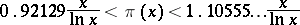for all sufficiently large. Actually,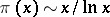, but this was not established until the end of the 19th century by means of complex analysis. For a long time it was considered impossible to obtain the result by elementary means. However, in 1949, A. Selberg obtained an elementary proof of this theorem. Chebyshev also proved that forthe interval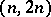contains at least one prime number. A refinement of the interval containing at least one prime number requires a deeper study of the behaviour of the function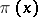.

In the 3rd century B.C. the sieve of Eratosthenes (cf. Eratosthenes, sieve of) was used to select the prime numbers from the set of natural numbers. In 1918 V. Brun showed that a modification of this method can serve as a basis for the study of a set of "almost primes" . He proved the Brun theorem on prime twins. The Brun sieve is a special case of general sieve methods (cf. Sieve method) which give estimates for collections of almost primes not exceedingand belonging to a sequenceof natural numbers. Sieve methods can be used when "good" approximations in the mean are known for the amount of integers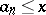belonging to a progression the modulus of which grows with. Among the sieve methods developed after Brun, the Selberg sieve is of special significance. The strongest results are obtained by a combination of sieve methods and analytic methods. A sieve method in conjunction with the Shnirel'man method made it possible to effectively find a numbersuch that any natural number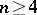can be represented as a sum of at mostprime numbers.

Two integersandare said to be congruent modulo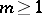if they have the same remainder on division by. Gauss (in 1801) introduced the notation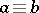. This form of writing, which brings about the analogy of congruences and equations, turned out to be convenient and instrumental in the development of the theory congruences (cf. Congruence).

Many results obtained previously by P. Fermat, L. Euler, J.L. Lagrange, and others, and also the Chinese remainder theorem, can be stated and proved simply in the language of the theory of congruences. One of the most interesting results of this theory is the quadratic reciprocity law.

The ancient Babylonians knew a large number of "Pythagorean triples" . Apparently they knew some method for finding arbitrary many integer solutions of the indeterminate equation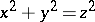. The Pythagoreans used the formulas,,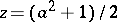to find solutions of this equation. Euclid indicated a method that allows one to find in succession integer solutions of the equation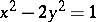(a special case of the Pell equation). In his Aritmetika Diophantus (3rd century B.C.) made an attempt to setting up a theory of indeterminate equations (see Diophantine equations). In particular, for the solution of equations of the second and some higher degrees he used systematically a device that enabled him to find from one rational solution of a given equation other rational solutions of it. Fermat (17th century) discovered another method, the "method of descent" , and solved by it a number of equations, but the so-called Fermat great theorem, which he declared to have solved, has turned out to be beyond the power of elementary methods.

Fermat solved the problem of representing natural numbers by sums of two squares of integers. As a result of research by Lagrange (1773) and Gauss (1801) the problem of the representation of integers by a definite binary quadratic form was solved. Gauss developed the general theory of binary quadratic forms. The solution of the problem of representing numbers by forms of higher degree (for example, the Waring problem) and by quadratic forms in several variables usually go beyond the framework of elementary methods. Only certain special cases of such problems can be solved elementarily. An example is Lagrange's theorem: Every natural number is the sum of four squares of integers. It should be mentioned that Diophantus in his Aritmetika repeatedly used the possibility of representing a natural number as the sum of four squares of integers.

To elementary number theory one also reckons the theory of partitions, the foundations of which were laid by Euler (in 1751). One of the basic problems of the theory of partitions is the study of the function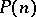, the number of representations of a natural numberas a sum of natural numbers. Other functions of similar type are also treated in the theory of partitions. Continued fractions (cf. Continued fraction) appeared in connection with problems of approximate computations (extraction of the square root of a natural number, search for approximations of real numbers by common fractions with small denominators). Continued fractions are applied in solving indefinite equations of the first and second degree. Using the apparatus of continued fractions J. Lambert (1766) was the first to establish that the numberis irrational. In the solution of various problems in the approximation of real numbers by rational numbers one uses in elementary number theory the Dirichlet principle, besides continued fractions.

In number theory it is easy to state many problems that can be formulated elementarily and have so far remained unsolved. For example; Is the set of even perfect numbers finite or not? Is there at least one odd perfect number? Is the set of Fermat prime numbers finite or not? Is the set of Mersenne prime numbers finite or not (cf. Mersenne number)? Is the set of prime numbers of the form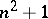finite or not? Is it true that there is at least one prime number between the squares of two consecutive natural numbers? Is the set of incomplete fractions of the expansion ofinto a continued fraction bounded or not?

How to Cite This Entry:
Elementary number theory. Encyclopedia of Mathematics. URL: http://encyclopediaofmath.org/index.php?title=Elementary_number_theory&oldid=13948
This article was adapted from an original article by A.A. BukhshtabV.I. Nechaev (originator), which appeared in Encyclopedia of Mathematics - ISBN 1402006098. See original article